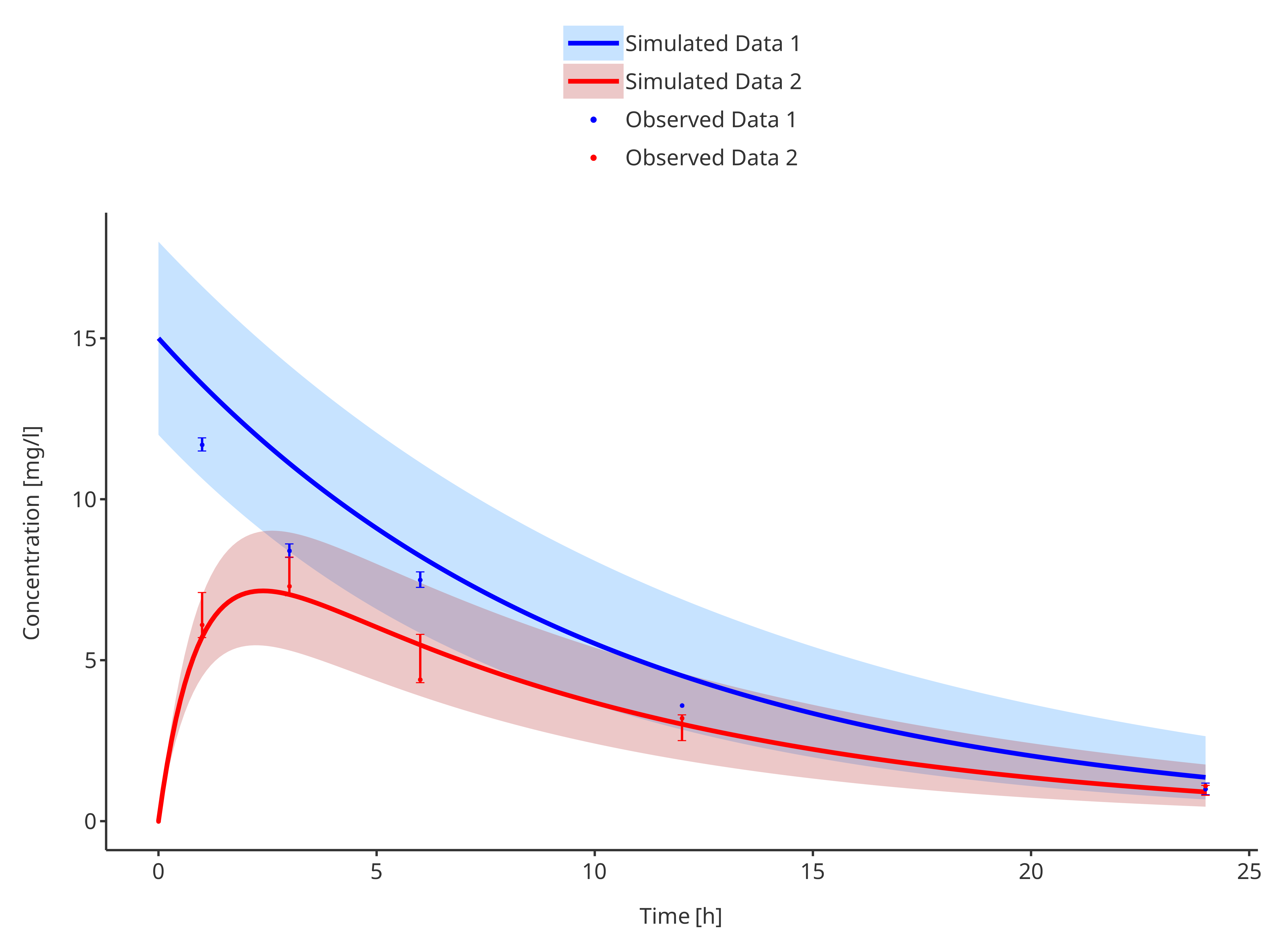require(tlf)
# Place default legend position above the plot for prettier time profile plots
setDefaultLegendPosition(LegendPositions$outsideTop) ## 1. Introduction The following vignette aims at documenting and illustrating workflows for producing Time Profile plots using the tlf-Library. Time profile plots aims at comparing observed and simulated data along time. In such plots, observed data are usually plotted as scatter points with errorbars showing population range or confidence intervals; while simulated data are usually plotted using lines with shaded ribbons showing population range or confidence intervals. ## 2. Definition of the time profile fonctions and classes ### 2.1. plotTimeProfile Besides, the usual tlf input arguments commonly used by the plot functions (data, metaData, dataMapping, plotConfiguration and plotObject), the function plotTimeProfile also includes the following optional input arguments: • observedData: A data.frame to use for plot. Unlike data, meant for simulated data, plotted as lines and ribbons; observedData is plotted as scatter points and errorbars. • observedDataMapping: An ObservedDataMapping object mapping x, y, ymin, ymax, and aesthetic groups to their variable names of observedData. ### 2.2. Data Mappings #### 2.2.1. dataMapping argument For time profile simulated data, TimeProfileDataMapping objects are required through the dataMapping argument of the function plotTimeProfile. As highlighted below, the class is initialized using the method $new. The optional arguments ymin and ymax define the shaded area of population range or confidence interval. The argument y is optional, only if ymin and ymax are provided.

It can be noted that the argument group can be used to group the data by these aesthetics.

TimeProfileDataMapping$new( x, y = NULL, ymin = NULL, ymax = NULL, group = NULL ) #### 2.2.2. observedDataMapping argument For time profile observed data, ObservedDataMapping objects are required through the observedDataMapping argument of the function plotTimeProfile. As highlighted below, the class is initialized using the method $new. The optional arguments ymin and ymax define the error bars of population range or confidence interval. The optional argument error can be used instead to calculate the errorbars from the variable y.

The argument mdv, meant for missing dependent variable, is a flag inspired from Nonmem for excluding data from the final time profile plot.

It can be noted that the argument group can be used to group the data by these aesthetics.

ObservedDataMapping$new( x, y, error = NULL, ymin = NULL, ymax = NULL, group = NULL, mdv = NULL ) #### 2.2.3. Remaining TODOs Some time profiles can also show another quantity in parallel and whose unit is different. A right axis is usually added for such plots to describe the values of that quantity. The TimeProfileDataMapping and ObservedData objects do not support yet a y2 argument for displaying data on the right axis. ## 3. Examples For the examples presented in the sequel, two simulated and two observed datasets are created below: # Simulation time simTime <- seq(0, 24, 0.1) # metaData will be used during the smart mapping # to label the axes as "dimension [unit]" metaData <- list( time = list( dimension = "Time", unit = "h" ), concentration = list( dimension = "Concentration", unit = "mg/l" ) ) # Spread metaData to min/max metaData$maxConcentration <- metaData$concentration metaData$minConcentration <- metaData$concentration # Simulated data 1: exponential decay simData1 <- data.frame( time = simTime, concentration = 15 * exp(-0.1 * simTime), minConcentration = 12 * exp(-0.12 * simTime), maxConcentration = 18 * exp(-0.08 * simTime), caption = "Simulated Data 1" ) # Simulated data 2: first order absorption with exponential decay simData2 <- data.frame( time = simTime, concentration = 10 * exp(-0.1 * simTime) * (1 - exp(-simTime)), minConcentration = 8 * exp(-0.12 * simTime) * (1 - exp(-simTime)), maxConcentration = 12 * exp(-0.08 * simTime) * (1 - exp(-simTime)), caption = "Simulated Data 2" ) # Observed data 1 obsData1 <- data.frame( time = c(1, 3, 6, 12, 24), concentration = c(11.7, 8.4, 7.5, 3.6, 1.0), sd = c(0.204, 0.21, 0.241, 0, 0.18), caption = "Observed Data 1" ) # Observed data 2 obsData2 <- data.frame( time = c(0, 1, 3, 6, 12, 24), concentration = c(0, 6.1, 7.3, 4.4, 3.2, 1.1), minConcentration = c(0, 5.7, 7.0, 4.3, 2.5, 0.8), maxConcentration = c(0, 7.1, 8.2, 5.8, 3.3, 1.11), caption = "Observed Data 2" ) The following sections show how to plot a Time Profile for specific scenarios ### 3.1. Plot simulated data only #### 3.1.1 Single simulation # Define Data Mapping simDataMapping <- TimeProfileDataMapping$new(
x = "time",
y = "concentration",
group = "caption"
)

plotTimeProfile(
data = simData1,
dataMapping = simDataMapping
)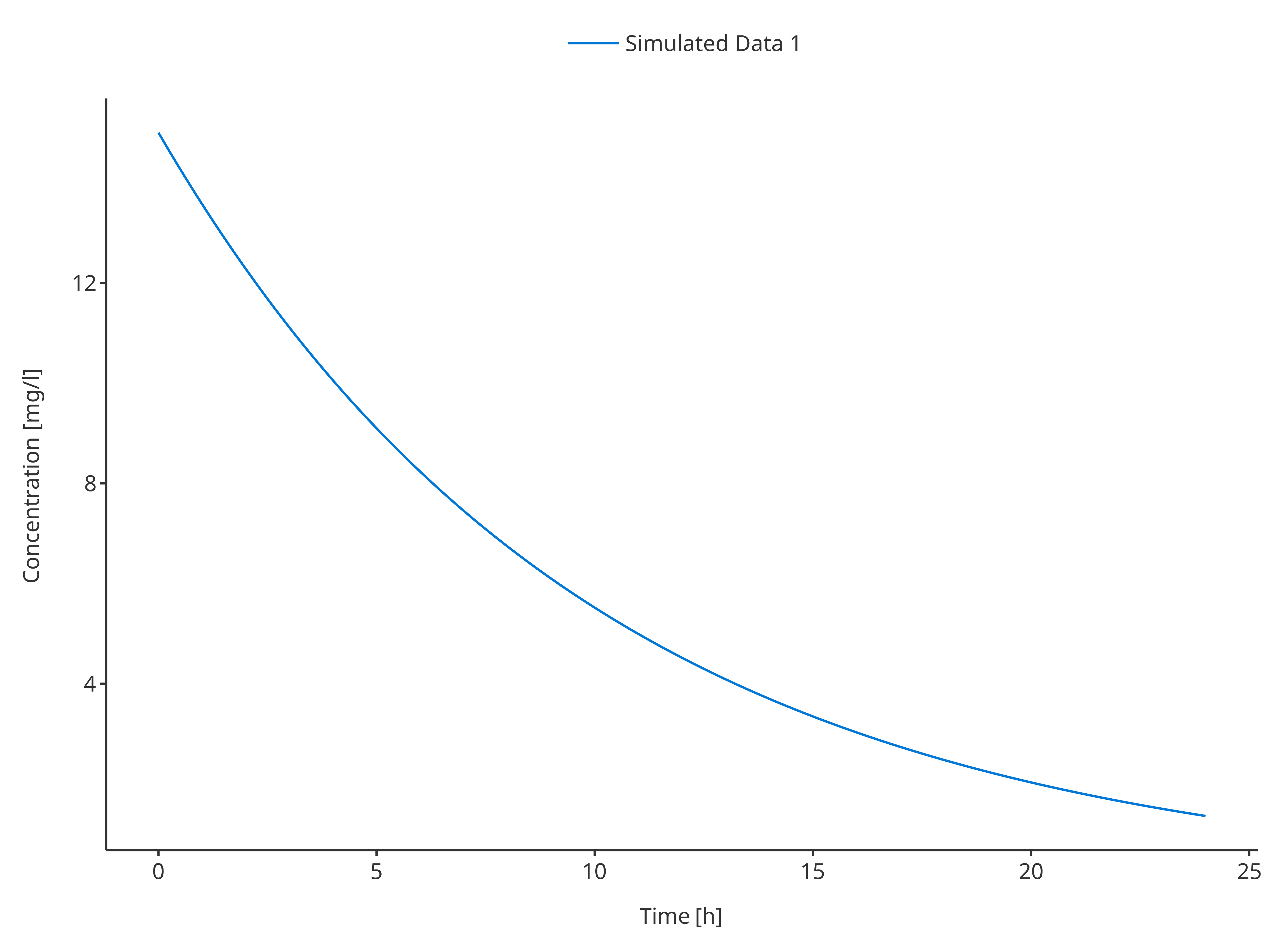plotTimeProfile(
data = simData2,
dataMapping = simDataMapping
)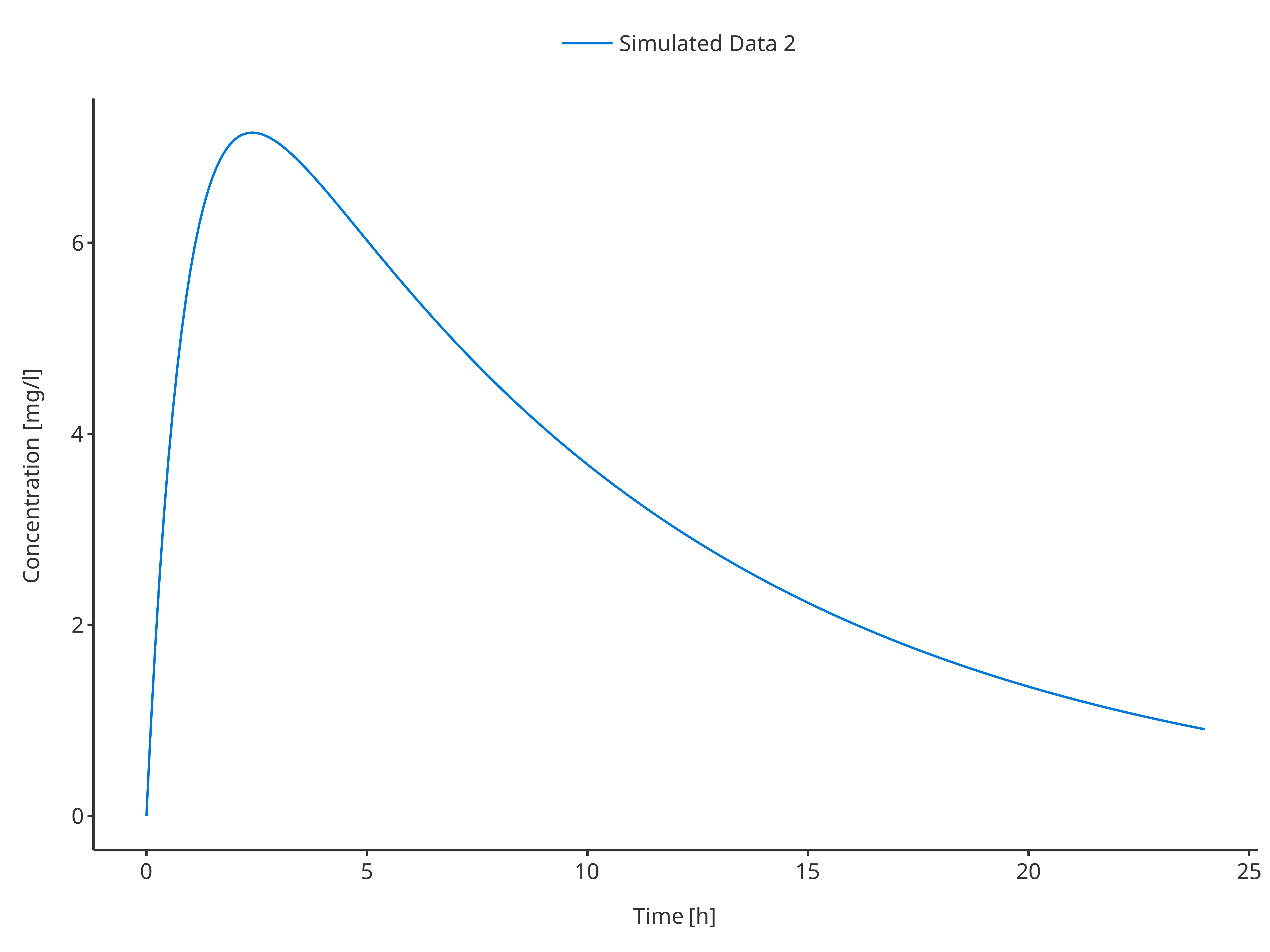#### 3.1.2. Single simulation with confidence interval

# Define Data Mapping
simDataMapping <- TimeProfileDataMapping$new( x = "time", y = "concentration", ymin = "minConcentration", ymax = "maxConcentration", group = "caption" ) plotTimeProfile( data = simData1, metaData = metaData, dataMapping = simDataMapping )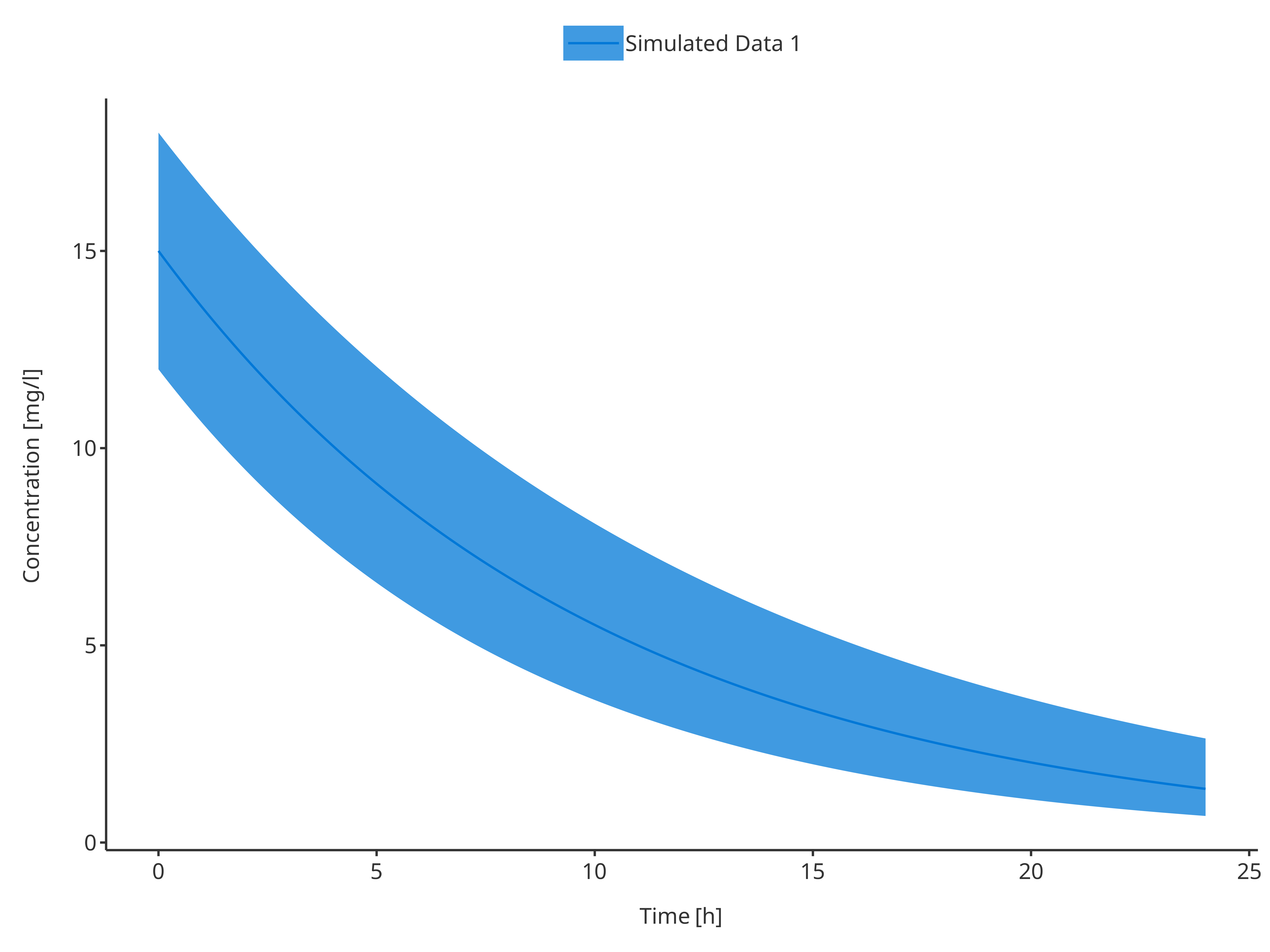plotTimeProfile( data = simData2, metaData = metaData, dataMapping = simDataMapping )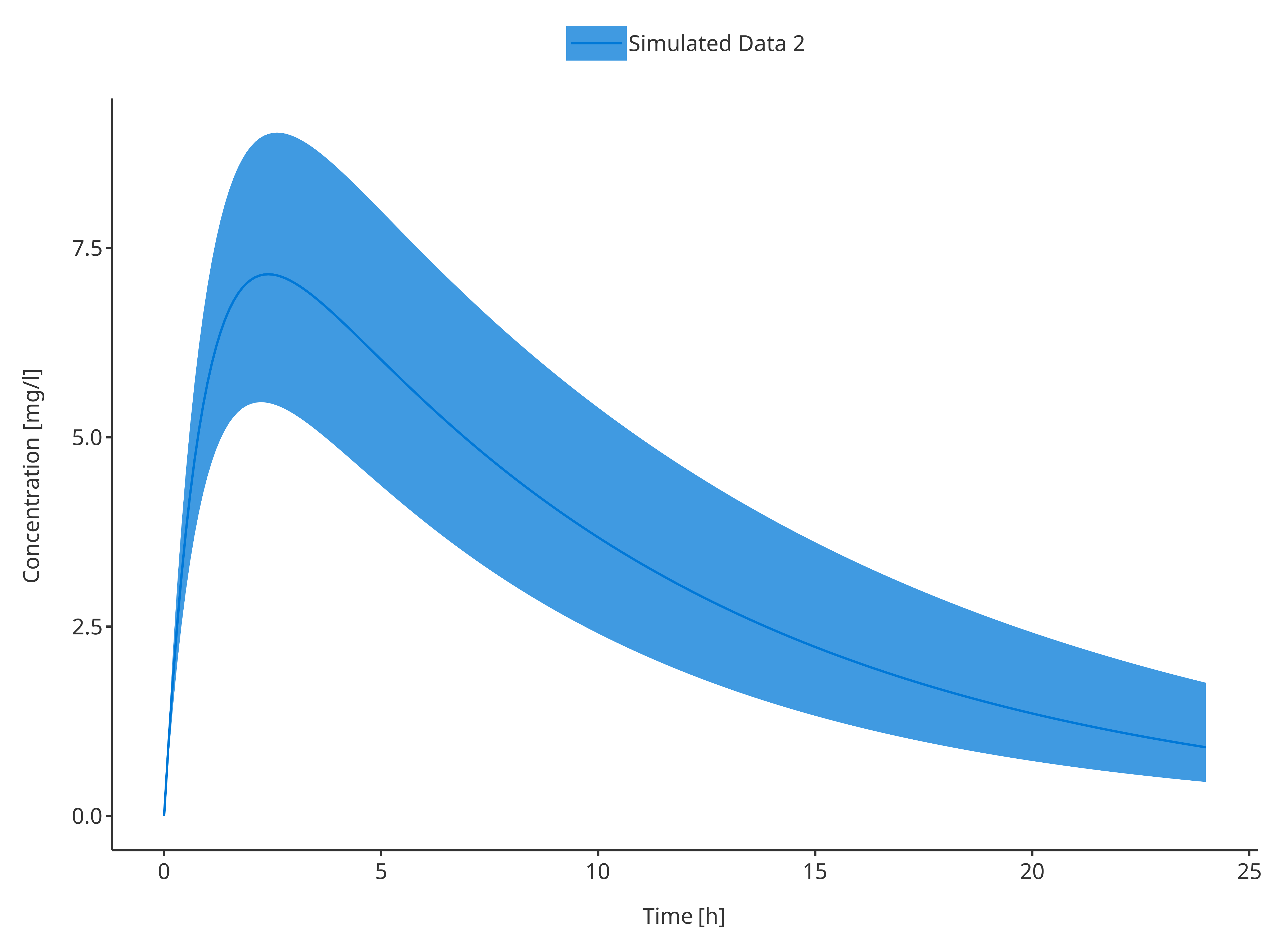#### 3.1.3. Multiple simulations # Define Data Mapping # Not using a group here will plot both profiles # but as they were the same data simDataMapping <- TimeProfileDataMapping$new(
x = "time",
y = "concentration"
)

plotTimeProfile(
data = rbind.data.frame(
simData1,
simData2
),
dataMapping = simDataMapping
)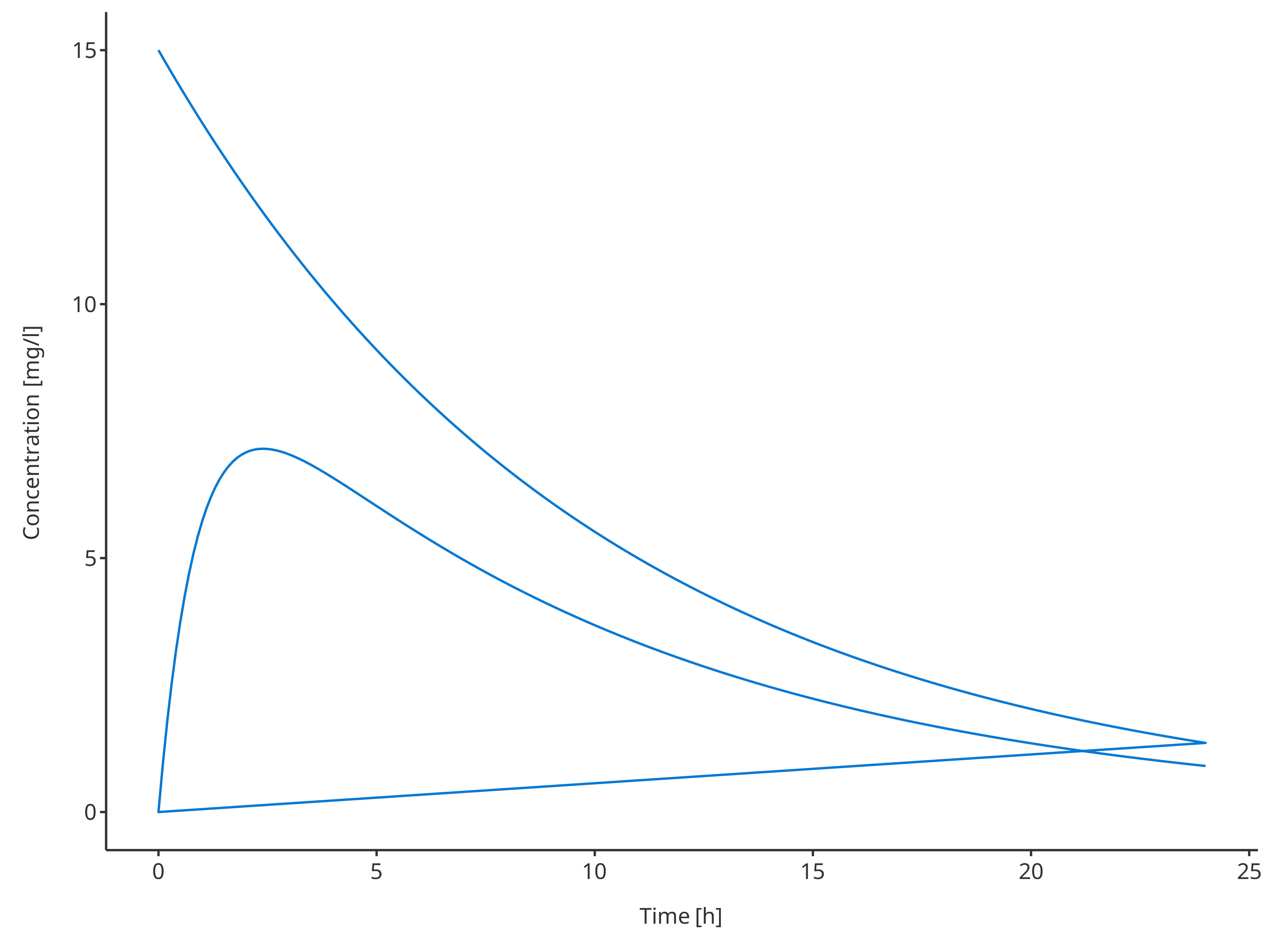# Define Data Mapping
# Using a group to plot both profiles

simDataMapping <- TimeProfileDataMapping$new( x = "time", y = "concentration", group = "caption" ) plotTimeProfile( data = rbind.data.frame( simData1, simData2 ), metaData = metaData, dataMapping = simDataMapping )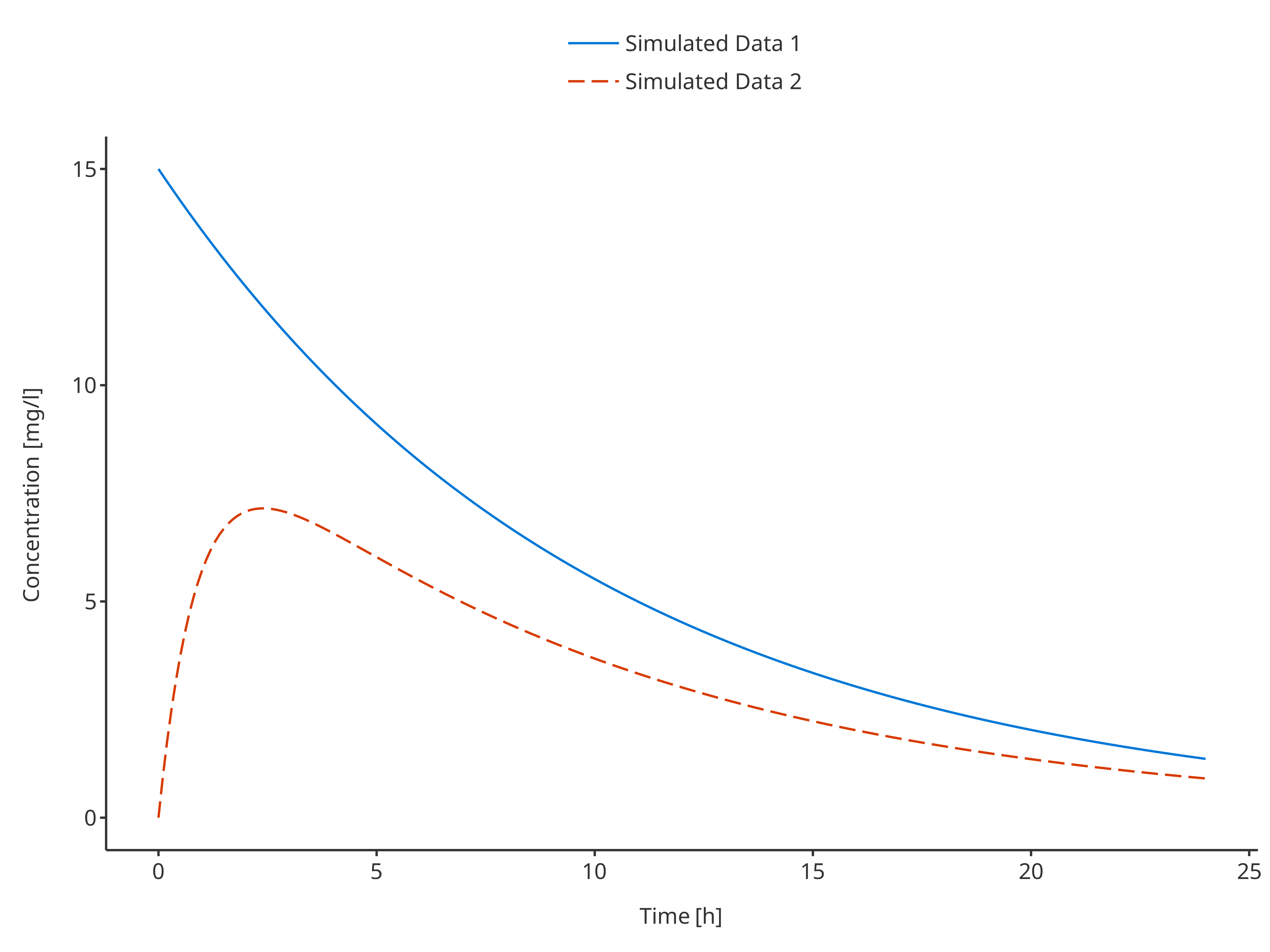#### 3.1.4. Multiple simulations with confidence interval # Define Data Mapping # ymin and ymax define the CI simDataMapping <- TimeProfileDataMapping$new(
x = "time",
y = "concentration",
ymin = "minConcentration",
ymax = "maxConcentration",
group = "caption"
)

plotTimeProfile(
data = rbind.data.frame(
simData1,
simData2
),
dataMapping = simDataMapping
)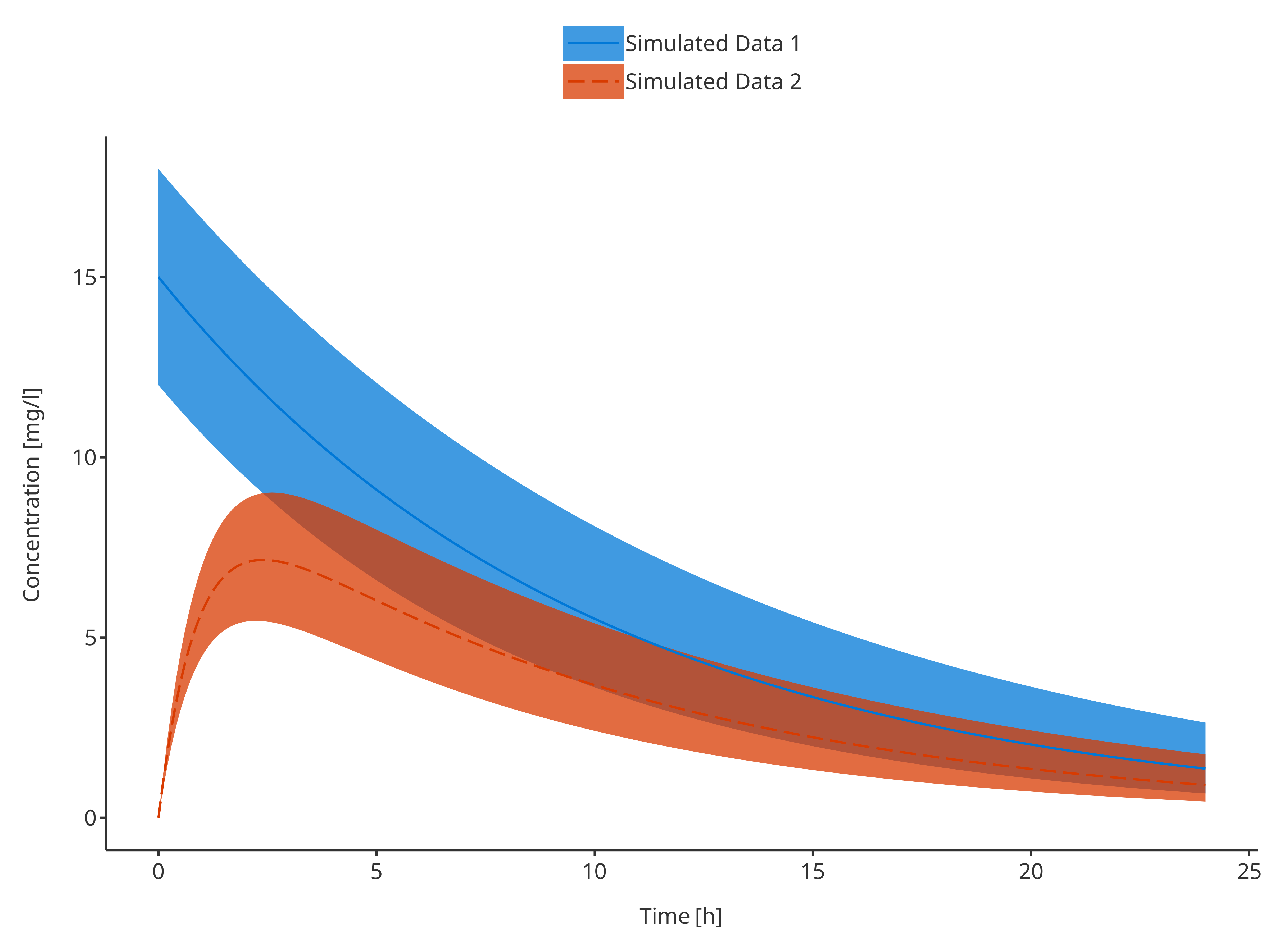### 3.2. Plot observed data only

#### 3.2.1. Single observed data set

# Define Data Mapping
obsDataMapping <- ObservedDataMapping$new( x = "time", y = "concentration", group = "caption" ) plotTimeProfile( observedData = obsData1, metaData = metaData, observedDataMapping = obsDataMapping )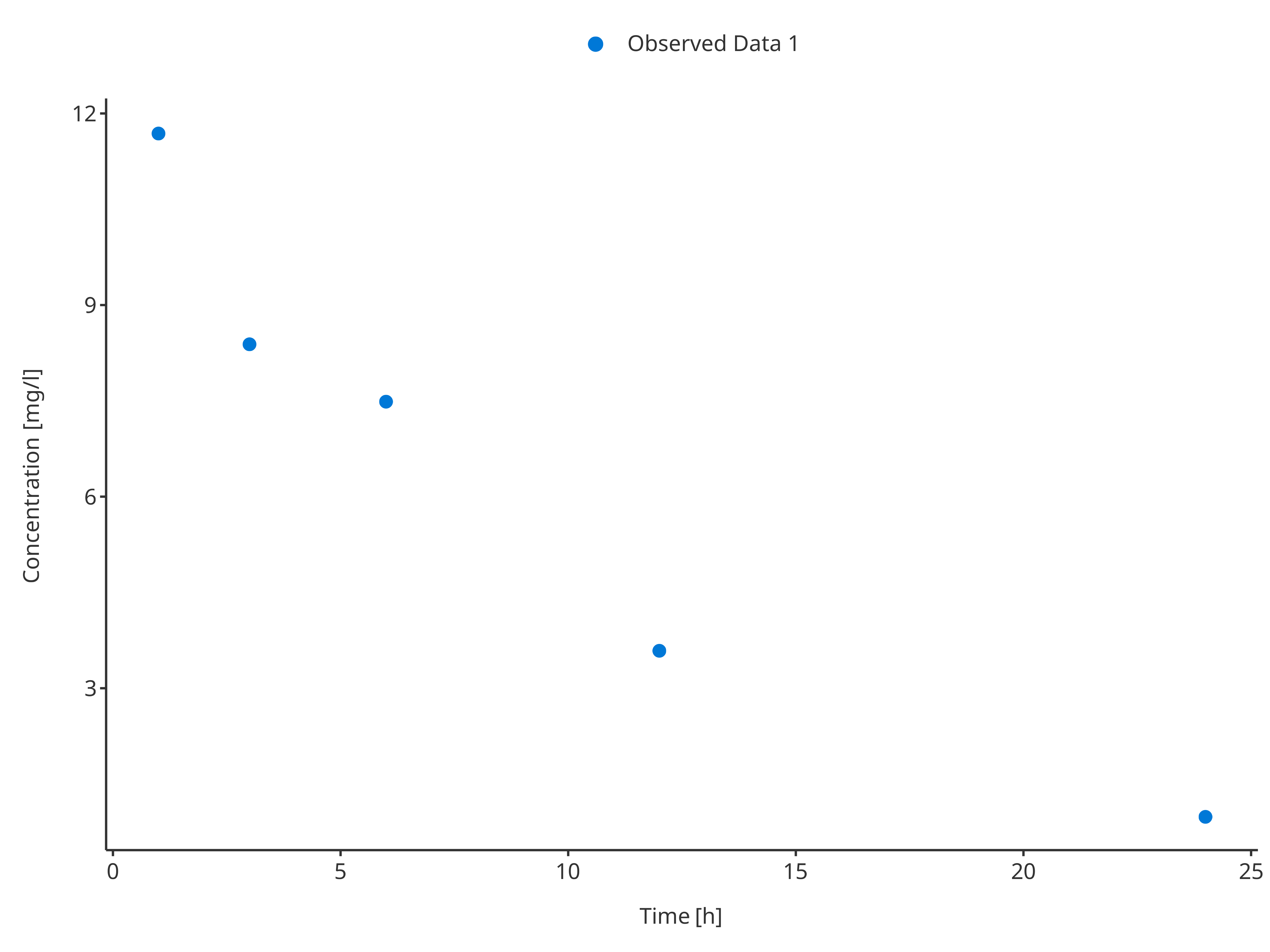plotTimeProfile( observedData = obsData2, metaData = metaData, observedDataMapping = obsDataMapping )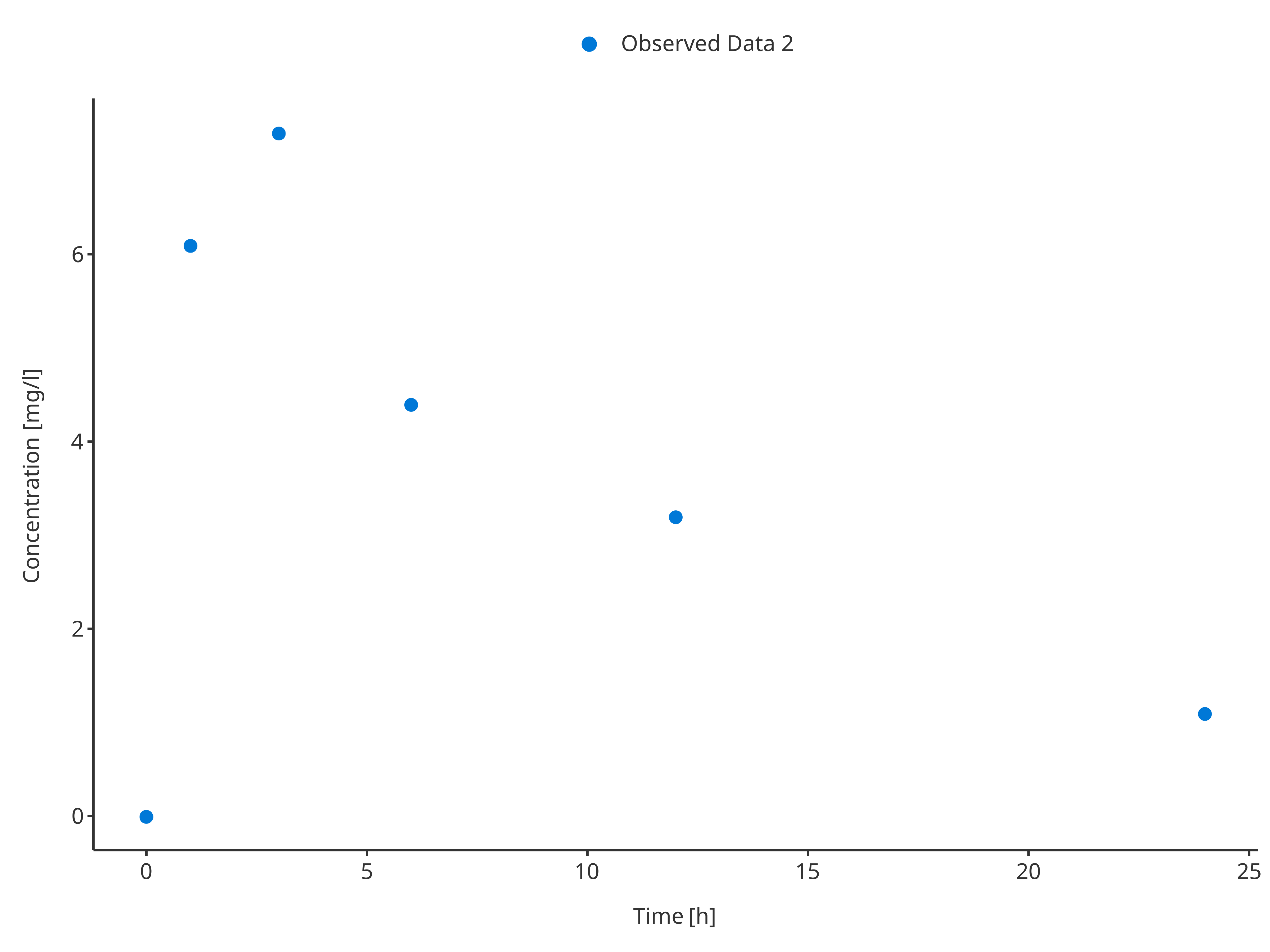#### 3.2.2. Single simulation with confidence interval For the first dataset, the uncertainty/error was defined as standard deviation using the variable "sd". This variable will be innerly used by the ObservedDataMapping object to create corresponding ymin and ymax values for the error bars. Tips • For data that include errorbars only partially, NA values cannot be used and should be replaced by 0 for error variable or y for ymin and ymax variables. • For logarithmic plots, negative ymin values of errorbars should be replaced by y beforehand in order to still see the ymax range of the errorbars. # Define Data Mapping obsDataMapping1 <- ObservedDataMapping$new(
x = "time",
y = "concentration",
error = "sd",
group = "caption"
)

plotTimeProfile(
observedData = obsData1,
observedDataMapping = obsDataMapping1
)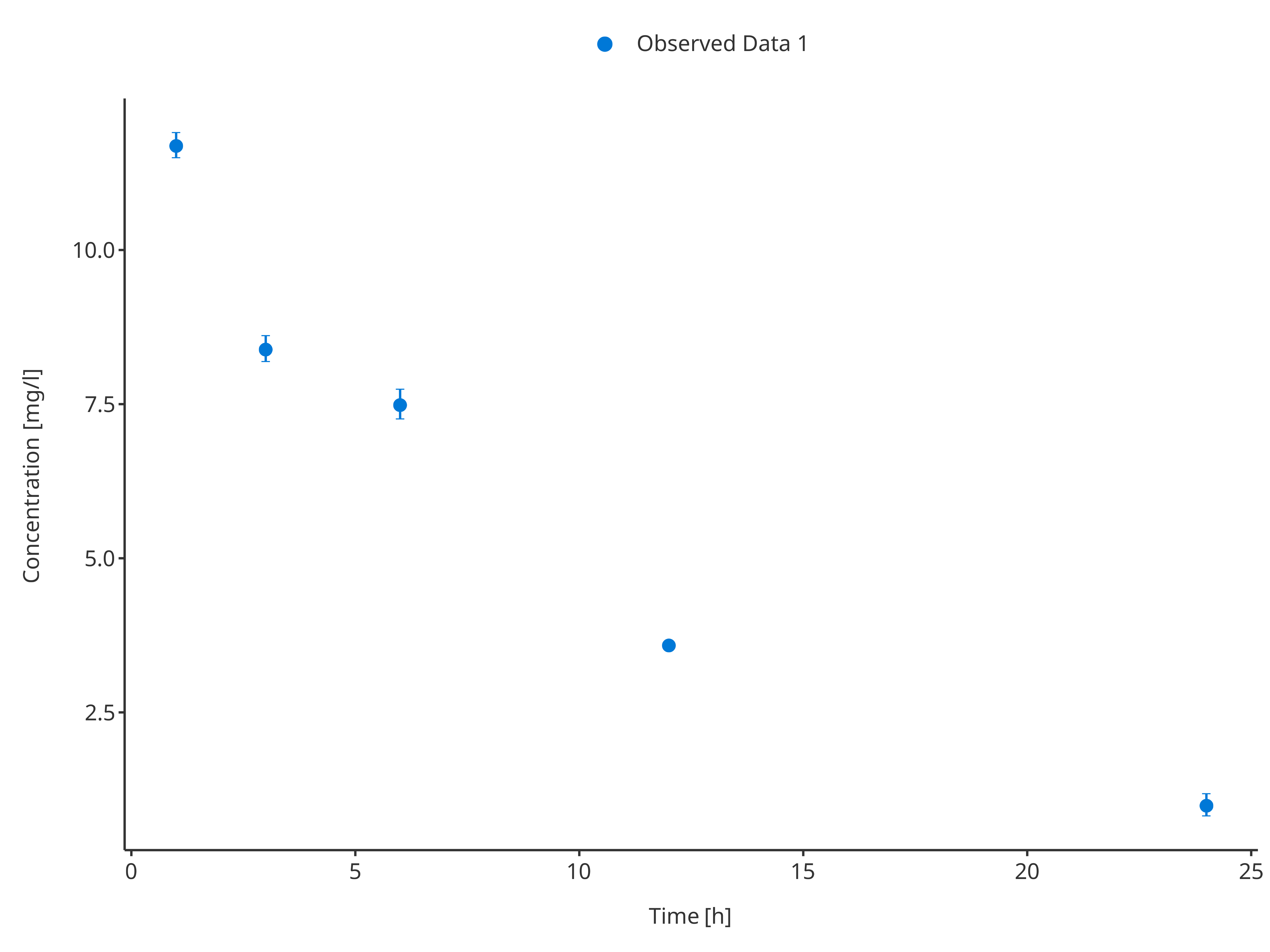For the second dataset, the uncertainty/error was defined as "minConcentration" and "minConcentration", they are input directly as ymin and ymax and will be taken as is by the ObservedDataMapping object.

# Define Data Mapping
obsDataMapping2 <- ObservedDataMapping$new( x = "time", y = "concentration", ymin = "minConcentration", ymax = "maxConcentration", group = "caption" ) plotTimeProfile( observedData = obsData2, metaData = metaData, observedDataMapping = obsDataMapping2 )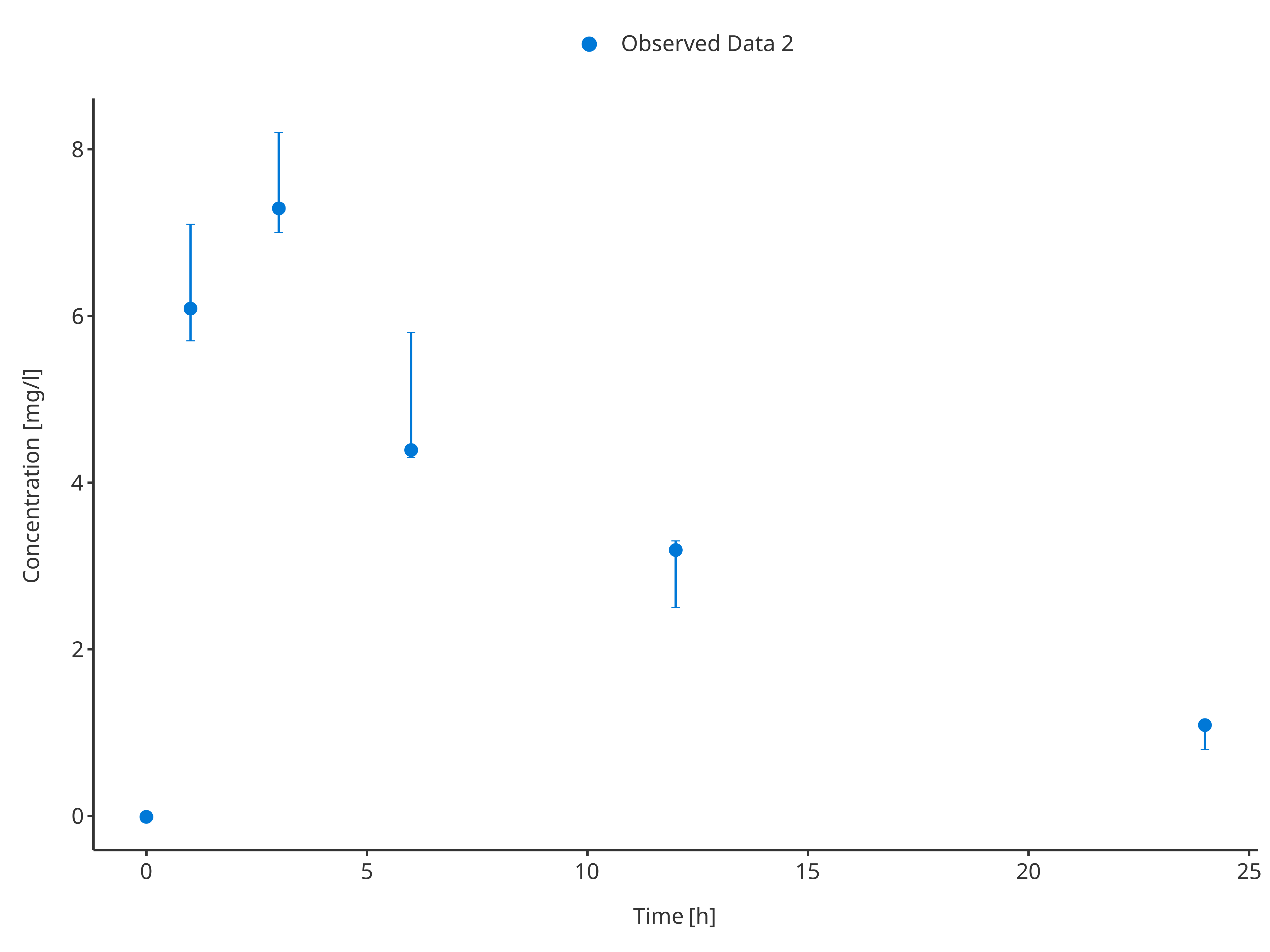#### 3.2.3. Multiple observed data sets # Define Data Mapping obsDataMapping <- ObservedDataMapping$new(
x = "time",
y = "concentration",
group = "caption"
)

plotTimeProfile(
observedData = rbind.data.frame(
obsData1[, c("time", "concentration", "caption")],
obsData2[, c("time", "concentration", "caption")]
),
observedDataMapping = obsDataMapping
)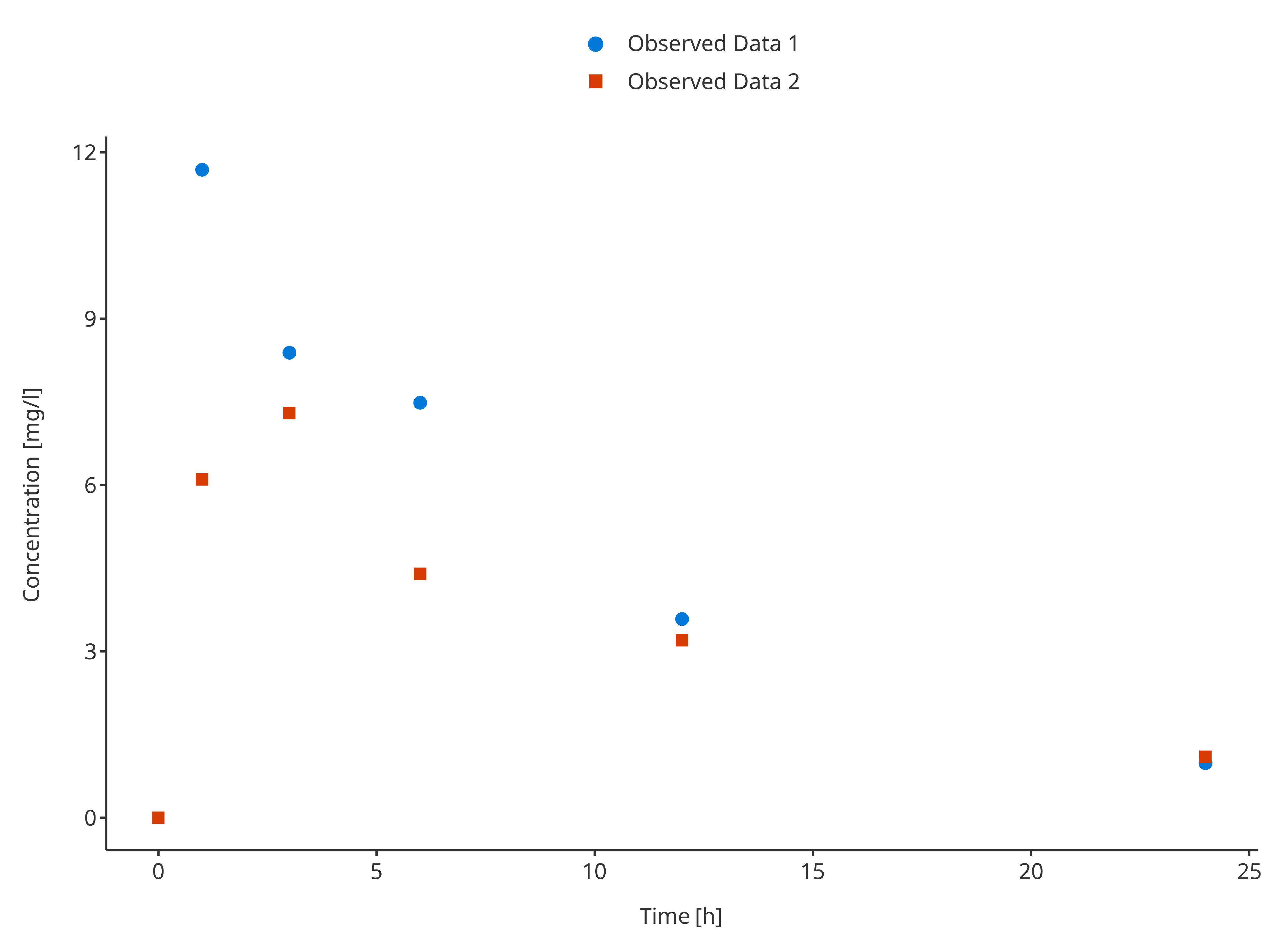#### 3.2.4. Multiple observed data sets with confidence interval

In this example, the observed data were not using the same way of displaying errors. Before plotting, the data needs to be merged in a consistent way to let the dataMapping know which variable(s) to use.

Below, ymin and ymax were consequently calculated for obsData1 before merging.

# Use common variable before usinf rbind.data.frame
obsData1$minConcentration <- obsData1$concentration - obsData1$sd obsData1$maxConcentration <- obsData1$concentration + obsData1$sd
obsData2$sd <- 0 # Define Data Mapping obsDataMapping <- ObservedDataMapping$new(
x = "time",
y = "concentration",
ymin = "minConcentration",
ymax = "maxConcentration",
group = "caption"
)

plotTimeProfile(
observedData = rbind.data.frame(
obsData1,
obsData2
),
observedDataMapping = obsDataMapping
)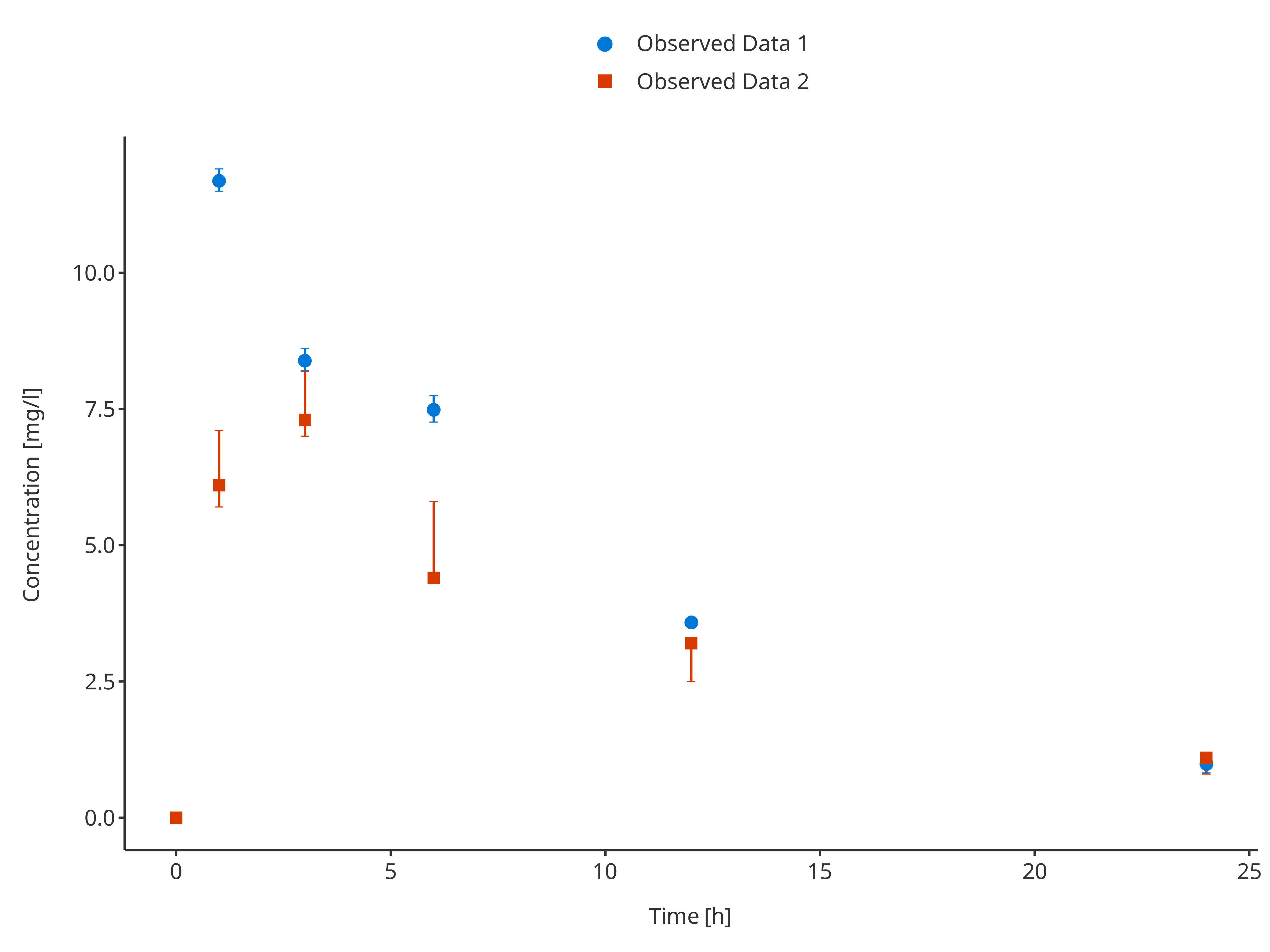#### 3.2.4. Missing Dependent Variable (mdv)

The following code flags all values higher than 10 as mdv, leadin to a plot without any observed data points higher than 10 (removing the first observation)

# Use common variable before usinf rbind.data.frame
mdvData <- obsData1
mdvData$mdv <- mdvData$concentration > 10

# Define Data Mapping
obsDataMapping <- ObservedDataMapping$new( x = "time", y = "concentration", ymin = "minConcentration", ymax = "maxConcentration", group = "caption", mdv = "mdv" ) plotTimeProfile( observedData = mdvData, metaData = metaData, observedDataMapping = obsDataMapping )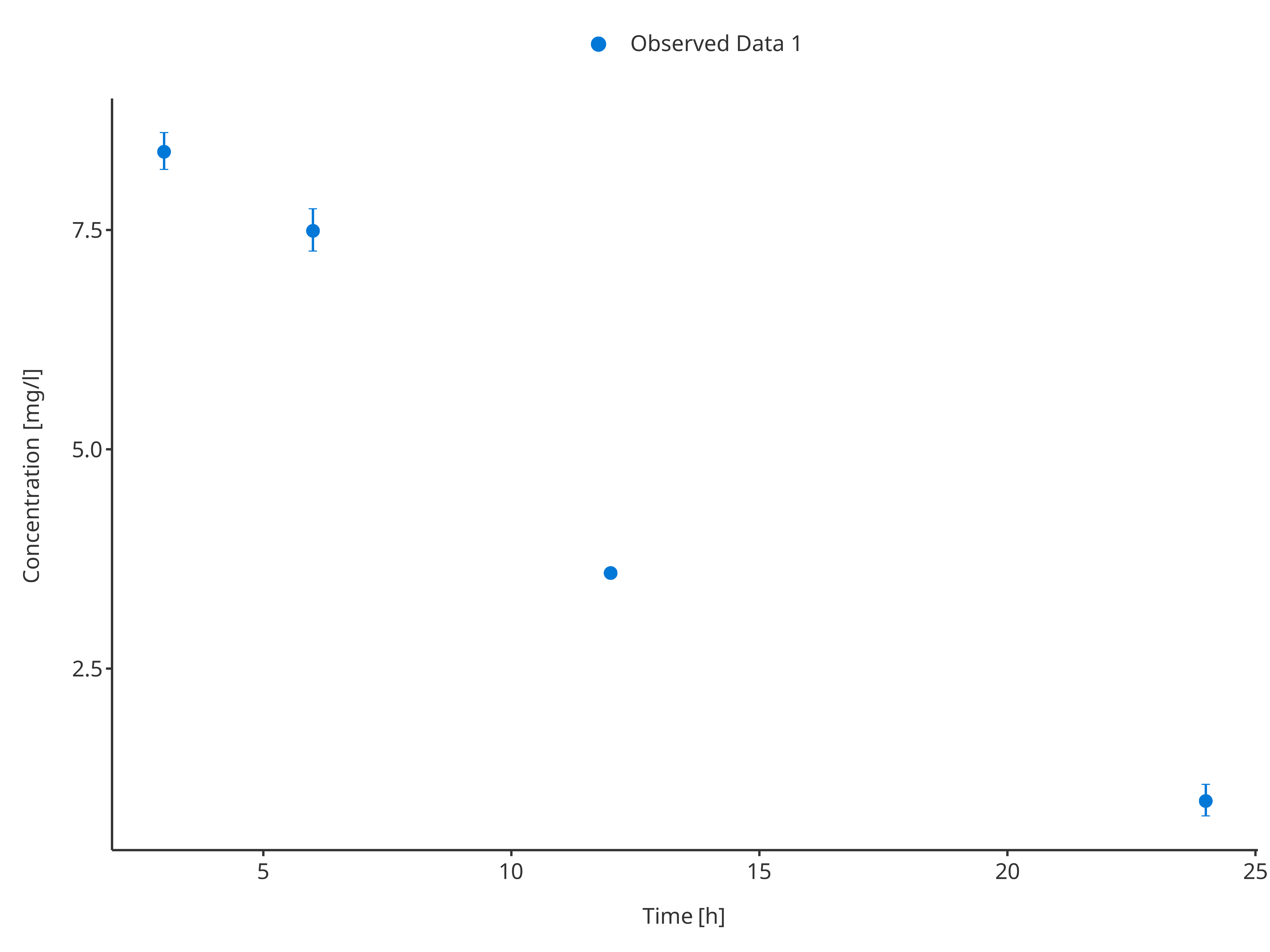### 3.3. Plot simulated and observed data By combining data and their dataMapping with observedData and their observedDataMapping, complex time profiles comparing observed and simulated data can be plotted. #### 3.3.1. Single simulation and observed data set # Define Data Mappings simDataMapping <- TimeProfileDataMapping$new(
x = "time",
y = "concentration",
group = "caption"
)

obsDataMapping <- ObservedDataMapping$new( x = "time", y = "concentration", group = "caption" ) plotTimeProfile( data = simData1, observedData = obsData1, metaData = metaData, dataMapping = simDataMapping, observedDataMapping = obsDataMapping )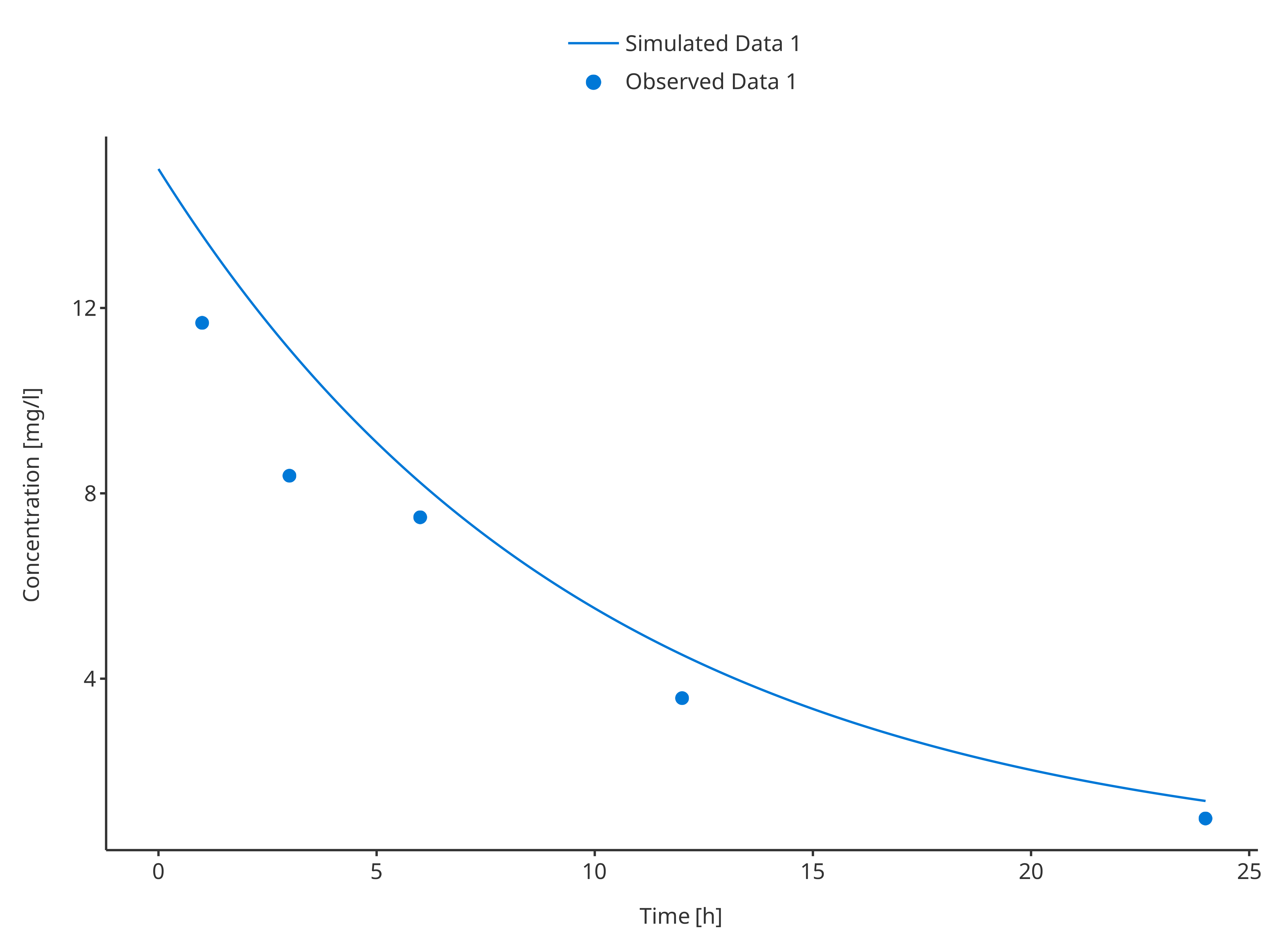plotTimeProfile( data = simData2, observedData = obsData2, metaData = metaData, dataMapping = simDataMapping, observedDataMapping = obsDataMapping )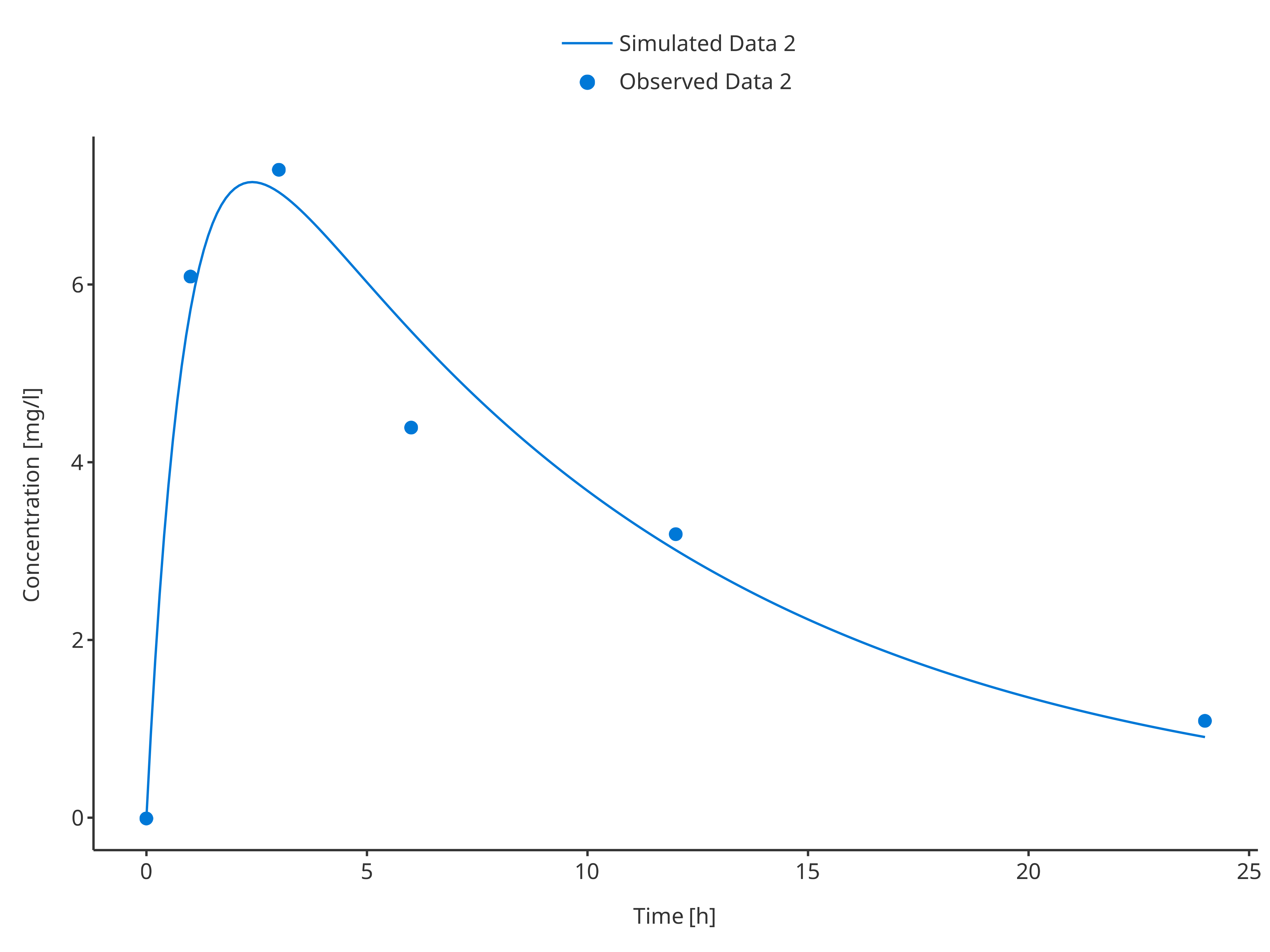#### 3.3.2. Single simulation and observed data set with their confidence intervals # Define Data Mappings simDataMapping <- TimeProfileDataMapping$new(
x = "time",
y = "concentration",
ymin = "minConcentration",
ymax = "maxConcentration",
group = "caption"
)

obsDataMapping <- ObservedDataMapping$new( x = "time", y = "concentration", ymin = "minConcentration", ymax = "maxConcentration", group = "caption" ) plotTimeProfile( data = simData1, observedData = obsData1, metaData = metaData, dataMapping = simDataMapping, observedDataMapping = obsDataMapping )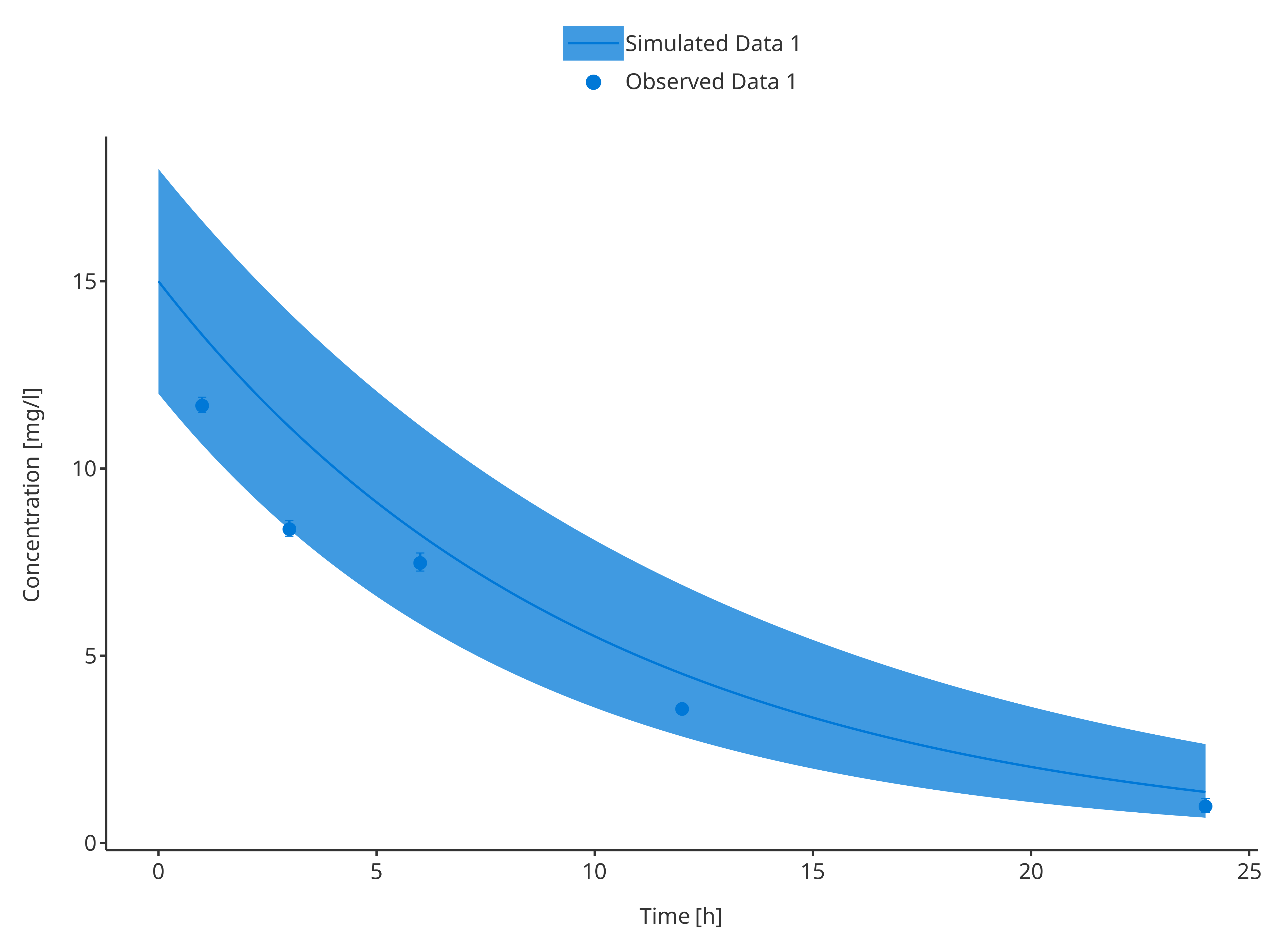plotTimeProfile( data = simData2, observedData = obsData2, metaData = metaData, dataMapping = simDataMapping, observedDataMapping = obsDataMapping )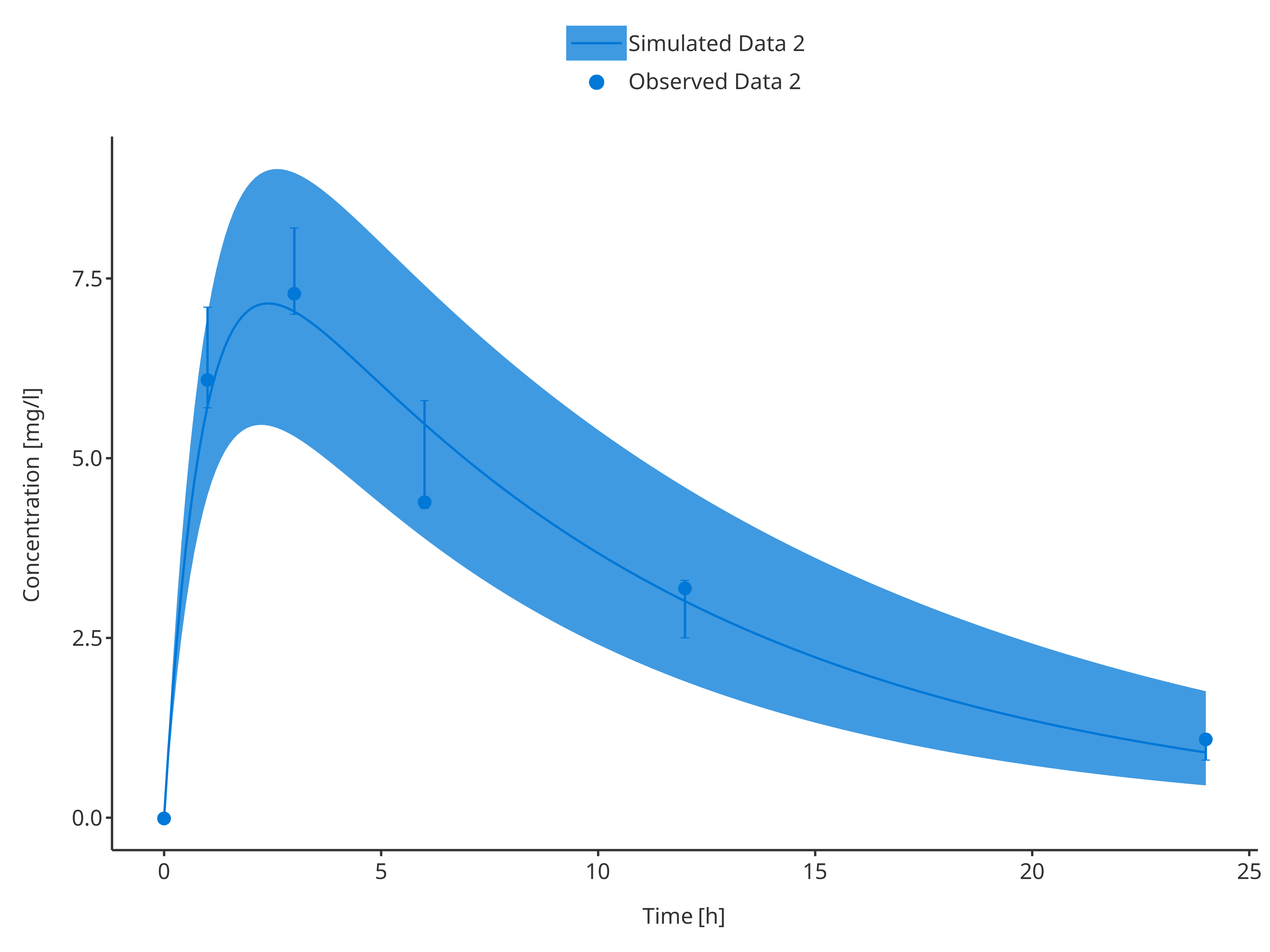#### 3.3.3. Mutliple simulations and observed data sets Combining multiple simulations and/or observed data sets is rather simple. To differentiate between the simulations/data sets, a grouping variable corresponding to the legend caption needs to be defined as illustrated below. # Define Data Mappings simDataMapping <- TimeProfileDataMapping$new(
x = "time",
y = "concentration",
group = "caption"
)

obsDataMapping <- ObservedDataMapping$new( x = "time", y = "concentration", group = "caption" ) simAndObsTimeProfile <- plotTimeProfile( data = rbind.data.frame( simData1, simData2 ), observedData = rbind.data.frame( obsData1, obsData2 ), metaData = metaData, dataMapping = simDataMapping, observedDataMapping = obsDataMapping ) simAndObsTimeProfile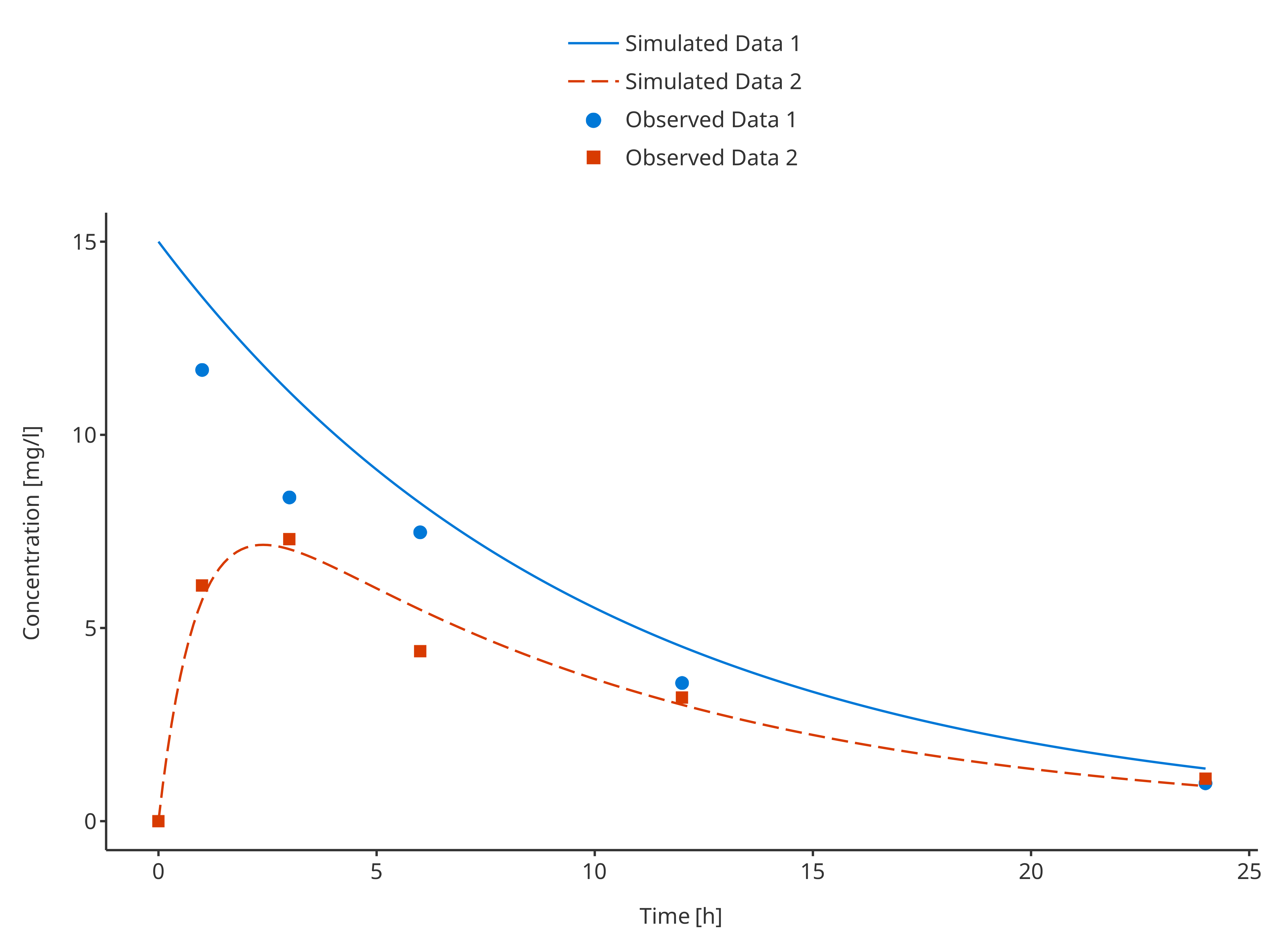In this example observed and simulated data do not share any mapping. Therefore, their color pattern may not match. To update the plot and have appropriate colors and labels in the legend, it is possible to get the legend properties and update them as illustrated in the example below that updates the positions of the legend entries: plotLegend <- getLegendCaption(simAndObsTimeProfile) knitr::kable(as.data.frame(plotLegend$color))
names labels values
Simulated Data 1 Simulated Data 1 #0078D7
Simulated Data 2 Simulated Data 2 #D83B01
Observed Data 1 Observed Data 1 #0078D7
Observed Data 2 Observed Data 2 #D83B01
plotLegend$color <- plotLegend$color[c(1, 3, 2, 4), ]
updateTimeProfileLegend(simAndObsTimeProfile, plotLegend)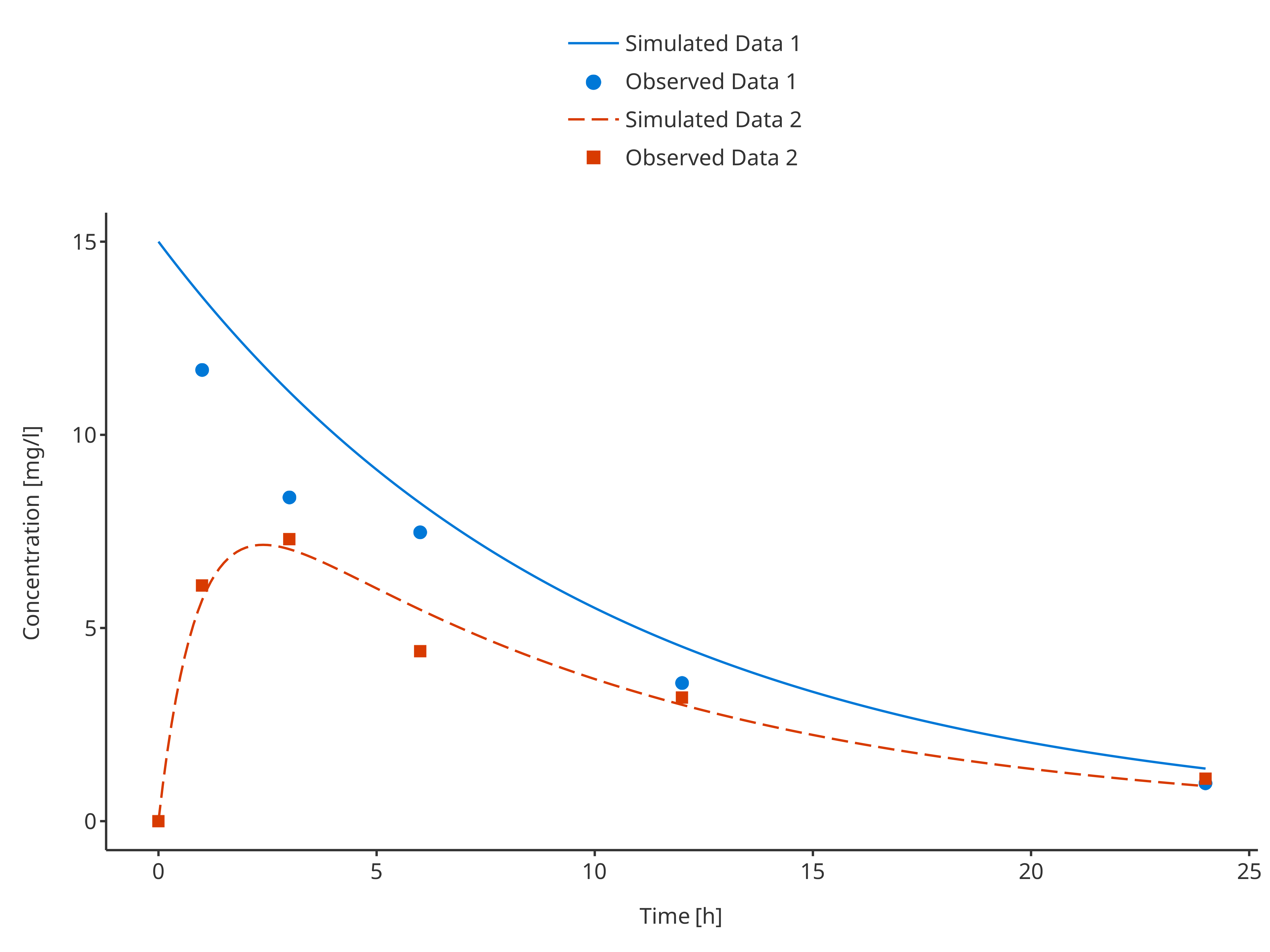Note that if observed and simulated data share common groupings, they will be merged in the final legend.

commonSimData1 <- simData1
commonObsData1 <- obsData1
commonSimData1$caption <- "Common data 1" commonObsData1$caption <- "Common data 1"
commonSimData2 <- simData2
commonObsData2 <- obsData2
commonSimData2$caption <- "Common data 2" commonObsData2$caption <- "Common data 2"

plotTimeProfile(
data = rbind.data.frame(commonSimData1, commonSimData2),
observedData = rbind.data.frame(commonObsData1, commonObsData2),
dataMapping = simDataMapping,
observedDataMapping = obsDataMapping
)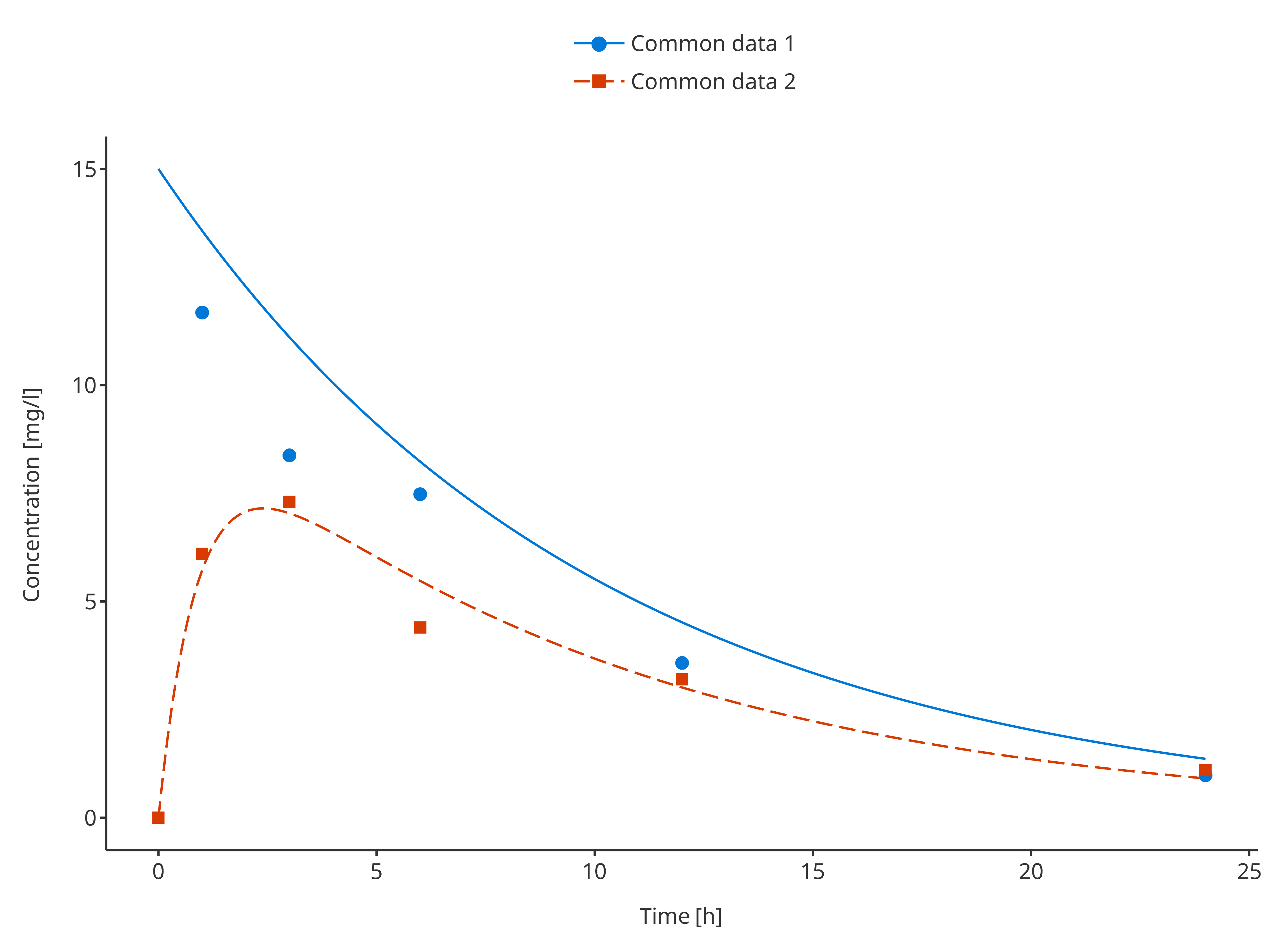#### 3.3.4. Mutliple simulations and observed data sets with their confidence intervals

# Define Data Mappings
simDataMapping <- TimeProfileDataMapping$new( x = "time", y = "concentration", ymin = "minConcentration", ymax = "maxConcentration", group = "caption" ) obsDataMapping <- ObservedDataMapping$new(
x = "time",
y = "concentration",
ymin = "minConcentration",
ymax = "maxConcentration",
group = "caption"
)

plotTimeProfile(
data = rbind.data.frame(
simData1,
simData2
),
observedData = rbind.data.frame(
obsData1,
obsData2
),
dataMapping = simDataMapping,
observedDataMapping = obsDataMapping
)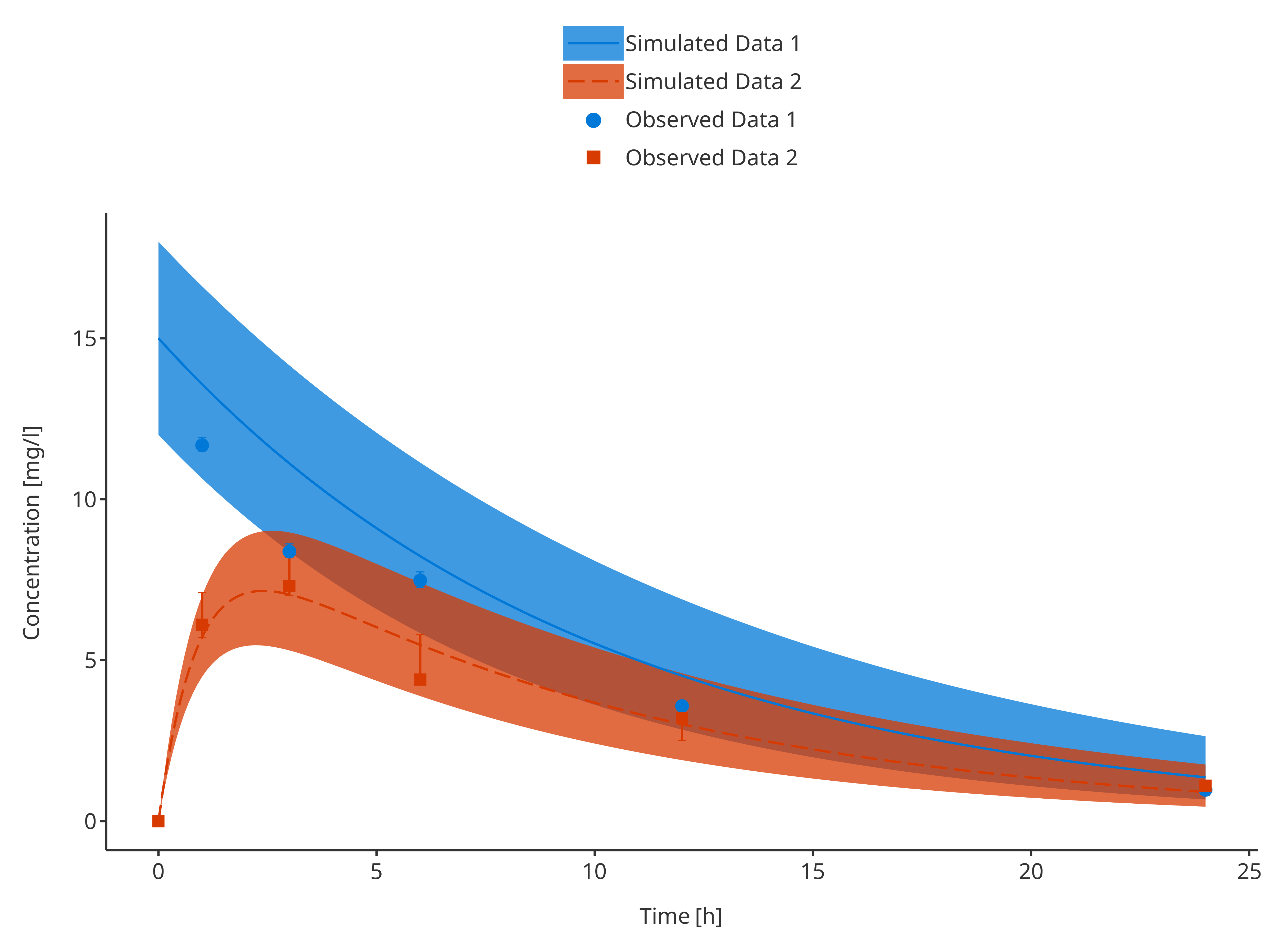### 3.4. Plot Configuration

For time profile plot properties can be defined in advance using TimeProfilePlotConfiguration objects.

The code below defines a smart plot configuration that uses data, metaData, and dataMapping to define the names of labels.

tpConfiguration <- TimeProfilePlotConfiguration$new( data = simData1, metaData = metaData, dataMapping = simDataMapping ) As every PlotConfiguration objects, TimeProfilePlotConfiguration objects include fields defining the properties of its lines, points, ribbons and errorbars. By default, these properties are used from the current theme, but can be overridden as illustrated below. # Define which lines are used for simulated data tpConfiguration$lines$linetype <- Linetypes$solid
tpConfiguration$lines$size <- 1
tpConfiguration$lines$color <- c("blue", "red")

# Define which ribbons are used for simulated data CI
tpConfiguration$ribbons$fill <- c("dodgerblue", "firebrick")
tpConfiguration$ribbons$alpha <- 0.25

# Define which shapes are used for observed data
tpConfiguration$points$shape <- Shapes$circle tpConfiguration$points$size <- 1 tpConfiguration$points$color <- c("blue", "red") # Define which errorbars are used for observed data CI tpConfiguration$errorbars$color <- "black" tpConfiguration$errorbars\$size <- 0.5

The corresponding plot is shown below:

plotTimeProfile(
data = rbind.data.frame(
simData1,
simData2
),
observedData = rbind.data.frame(
obsData1,
obsData2
),
)